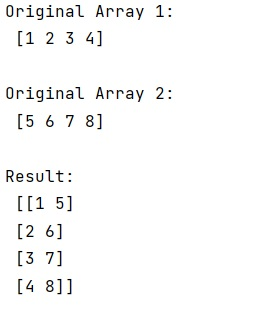# How to zip two 1d numpy array to 2d numpy array?

Learn, how to zip two 1d numpy array to 2d numpy array in Python?
Submitted by Pranit Sharma, on January 20, 2023

NumPy is an abbreviated form of Numerical Python. It is used for different types of scientific operations in python. Numpy is a vast library in python which is used for almost every kind of scientific or mathematical operation. It is itself an array which is a collection of various methods and functions for processing the arrays.

## Zipping two 1d numpy array to 2d numpy array

Suppose we are given two NumPy 1d arrays and we need to get a 2d array that contains both of these 1d arrays.

For very small arrays, zip() function can be faster than NumPy functions but in the case of longer arrays numpy functions are much faster.

Hence, for this purpose, the numpy.column_stack() method. This method stack 1-D arrays as columns into a 2-D array. Take a sequence of 1-D arrays and stack them as columns to make a single 2-D array. 2-D arrays are stacked as-is, just like with hstack. 1-D arrays are turned into 2-D columns first.

Let us understand with the help of an example,

## Python code to zip two 1d numpy array to 2d numpy array

```# Import numpy
import numpy as np

# Creating two numpy arrays
arr1 = np.array([1,2,3,4])
arr2 = np.array([5,6,7,8])

# Display original arrays
print("Original Array 1:\n",arr1,"\n")
print("Original Array 2:\n",arr2,"\n")

# Combining both arrays to create a 2d array
res = np.column_stack((arr1,arr2))

# Display the result
print("Result:\n",res)
```

Output:What's New (MCQs)

Top Interview Coding Problems/Challenges!

IncludeHelp's Blogs

Languages: » C » C++ » C++ STL » Java » Data Structure » C#.Net » Android » Kotlin » SQL
Web Technologies: » PHP » Python » JavaScript » CSS » Ajax » Node.js » Web programming/HTML
Solved programs: » C » C++ » DS » Java » C#
Aptitude que. & ans.: » C » C++ » Java » DBMS
Interview que. & ans.: » C » Embedded C » Java » SEO » HR
CS Subjects: » CS Basics » O.S. » Networks » DBMS » Embedded Systems » Cloud Computing
» Machine learning » CS Organizations » Linux » DOS
More: » Articles » Puzzles » News/Updates